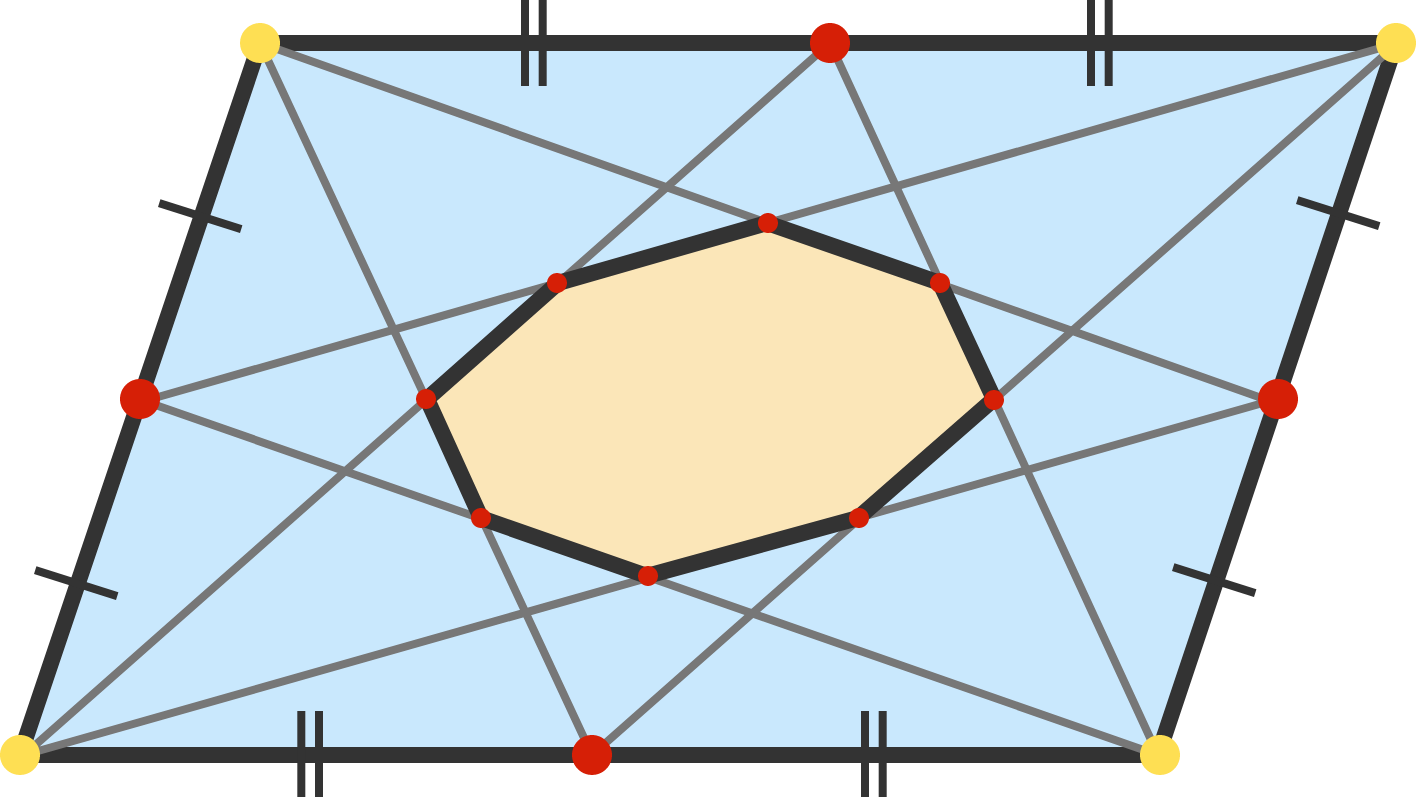# Octo-parallelogram!

Geometry Level 2Given any parallelogram, draw two line segments from each corner to connect to the midpoints of the opposite sides. These eight line segments form an octagon, as shown above.

Let $O$ be the octagon's area and let $P$ be the parallelogram's area. To three decimal places, what is $\frac{O}{P}?$

×